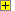Zener Diode
Voltage RegulatorThe power supply shown below uses a   V rated zener diode to give a regulated output of   V from an input voltage of   V
The zener diode must conduct at least   mA
The maximum power supply output current required is   mA
Each question is out of 100 marks. Marks are lost for using  help and if you get any part wrong. Enjoy yourself.
Zener diode as
a voltage regulator

Vin =   V
Vout =   V
minimum current through zener diode (D1) =   mA
maximum power supply output current =   mA

Calculate: (answers to 1dp or integers as appropriate)
 * the maximum current throught resistor (R1) = mA * the voltage drop across R1 = V * the value of R1 under these conditions = Ω
The current drawn from the power supply falls to zero.

Calculate: (answers to 1dp or integers as appropriate)
 * the current now flowing through the zener diode = mA * the power now dissipated by the zener diode = mWShow Calculator